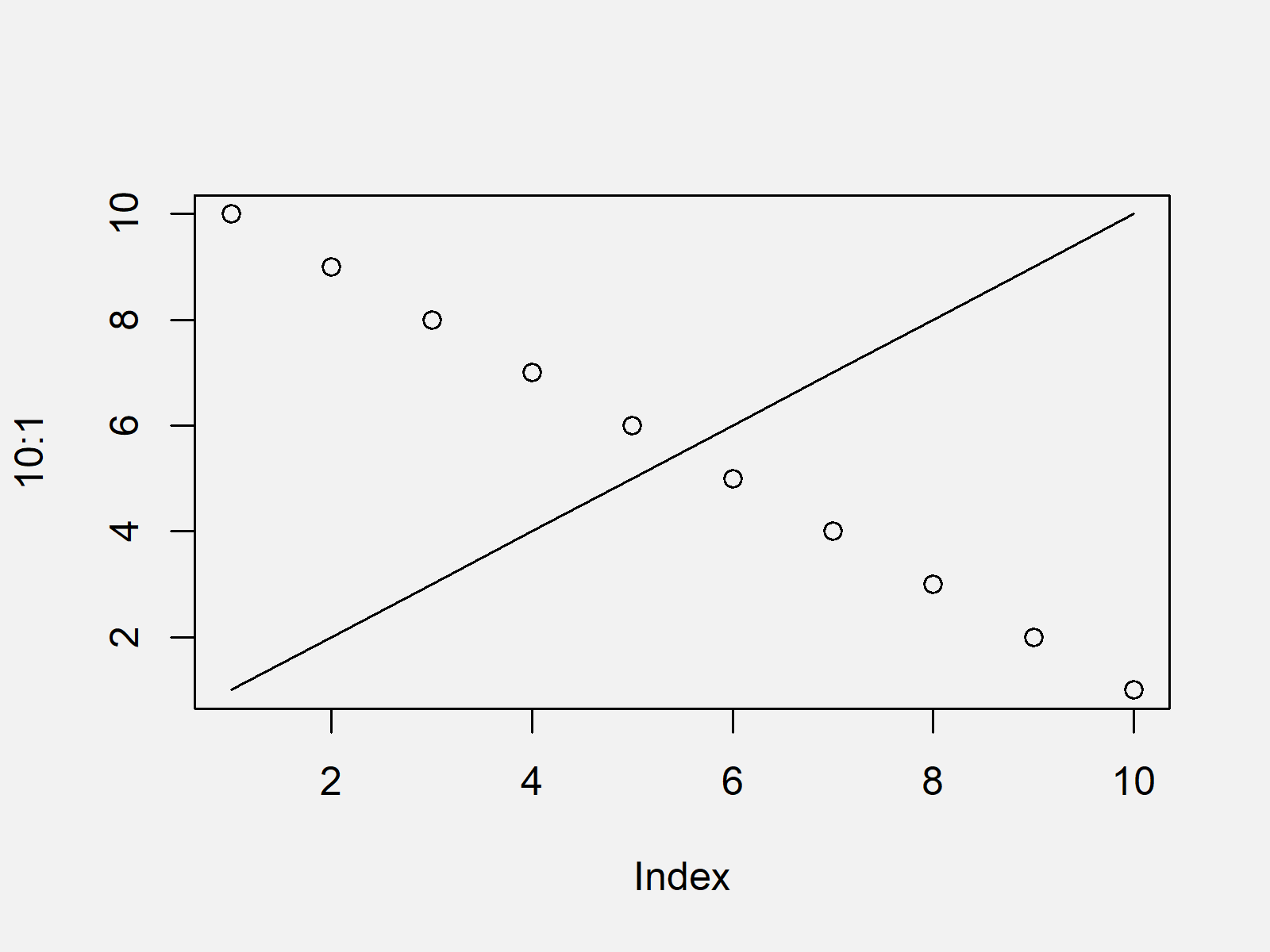# R Error: plot.new has not been called yet (2 Examples)

In this R programming article you’ll learn how to solve the error “plot.new has not been called yet”.

The page looks as follows:

It’s time to dive into the exemplifying R code:

## Example 1: Reproduce the Error: plot.new has not been called yet

Example 1 illustrates how to replicate the error message “plot.new has not been called yet” in R.

Let’s assume that we want to draw a line to a plot. Then, we might try to use the lines function as shown below:

```lines(1:10) # Try to add lines to non-existent plot # Error in plot.xy(xy.coords(x, y), type = type, ...) : # plot.new has not been called yet```

Unfortunately, the RStudio console returns the error message “plot.new has not been called yet”.

The reason for this is that some functions require that a plot was already created before their application (the lines function is one of them).

Next, I’ll show you how to solve this problem.

## Example 2: Fix the Error: plot.new has not been called yet

Example 2 illustrates how to fix the error message “plot.new has not been called yet”.

As explained in Example 1, we first have to draw a graphic before we can apply the lines function. Have a look at the following R code:

```plot(10:1) # Draw plot lines(1:10) # Add lines to plot```As shown in Figure 1, we created a scatterplot containing a line with the previous R programming syntax.

## Video, Further Resources & Summary

Have a look at the following video of my YouTube channel. I illustrate the R code of this tutorial in the video.

Besides the video, you could have a look at the related articles of this website:

This tutorial explained how to handle the error message “plot.new has not been called yet” in the R programming language.

In this tutorial, we have used the lines function to illustrate the error message. Please note that other functions such as abline lead to the same (or very similar) error messages, when a plot has not been created before these functions are called.